# CPSC 320 Intermediate Algorithm Design and Analysis July

• Slides: 18
Download presentation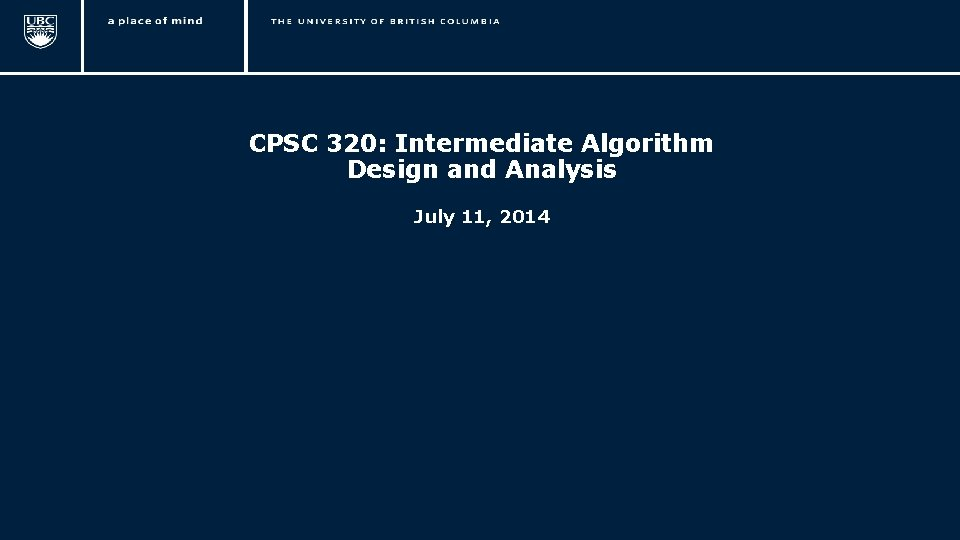CPSC 320: Intermediate Algorithm Design and Analysis July 11, 2014 1Course Outline • • • Introduction and basic concepts Asymptotic notation Greedy algorithms Graph theory Amortized analysis Recursion Divide-and-conquer algorithms Randomized algorithms Dynamic programming algorithms NP-completeness 2Reviewing some proof concepts 3Concepts related to proofs • • • Hypothesis: what we assume is true Proposition: what we want to prove is true Definition: something that we name, that doesn’t need a proof Theorem: something that is not evident that we provide a proof Lemma: an intermediary step in a proof (a “mini-theorem”) Corollary: something that can be inferred from previous results very easily 4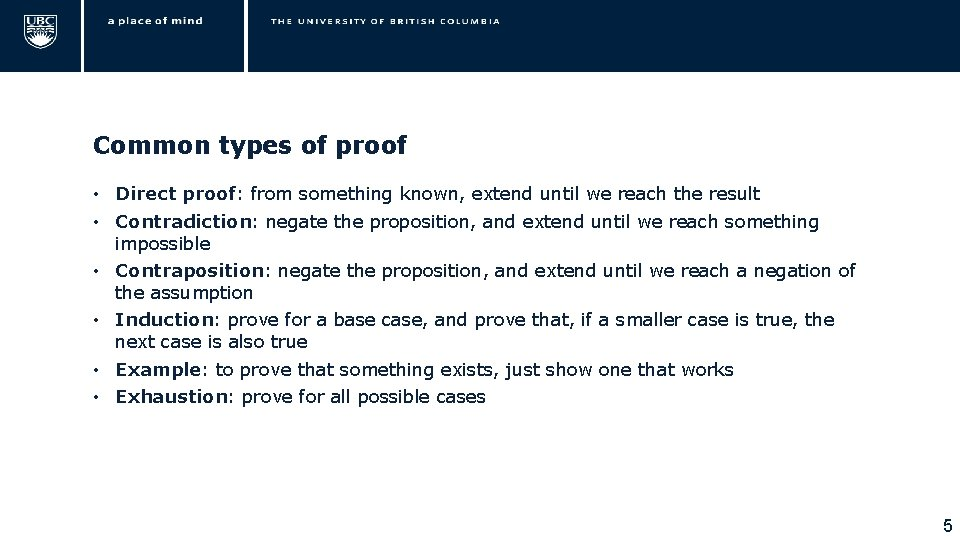Common types of proof • Direct proof: from something known, extend until we reach the result • Contradiction: negate the proposition, and extend until we reach something impossible • Contraposition: negate the proposition, and extend until we reach a negation of the assumption • Induction: prove for a base case, and prove that, if a smaller case is true, the next case is also true • Example: to prove that something exists, just show one that works • Exhaustion: prove for all possible cases 5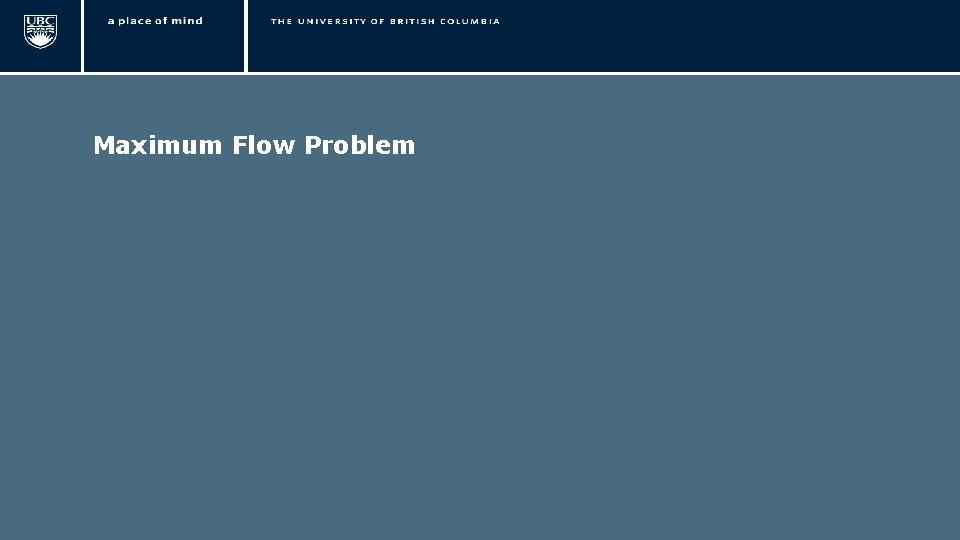Maximum Flow Problem 6Maximum Flow • Assume a directed graph where each edge has a capacity • Assume two nodes (source and sink) • Problem: find a flow from source to sink that uses maximum possible value • In any node (except source and sink) incoming flow equals outgoing flow • Application: • Flow of materials from a system to a client (e. g. , water pipes) • Flow of electrical current through a network • Transportation of goods 7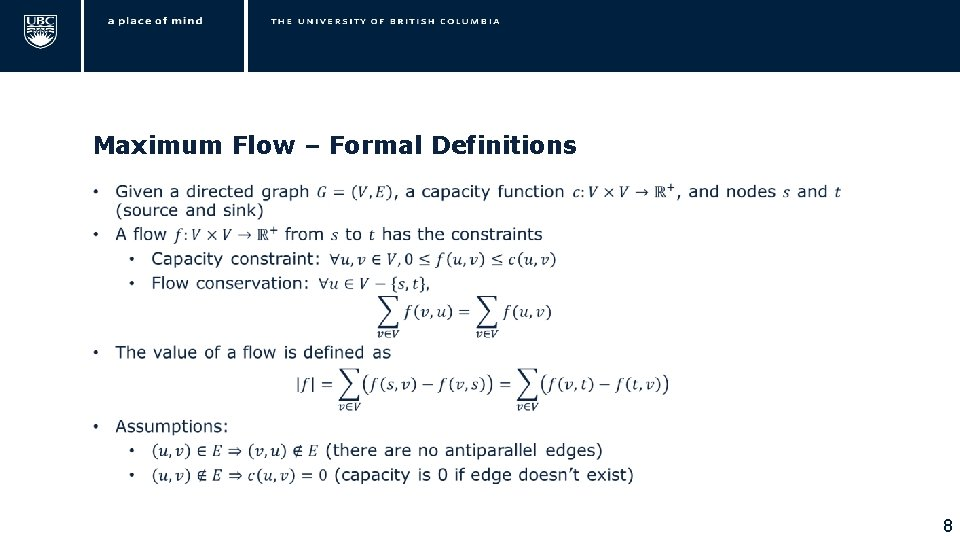Maximum Flow – Formal Definitions • 8Ford-Fulkerson Method • 9Residual Network • 10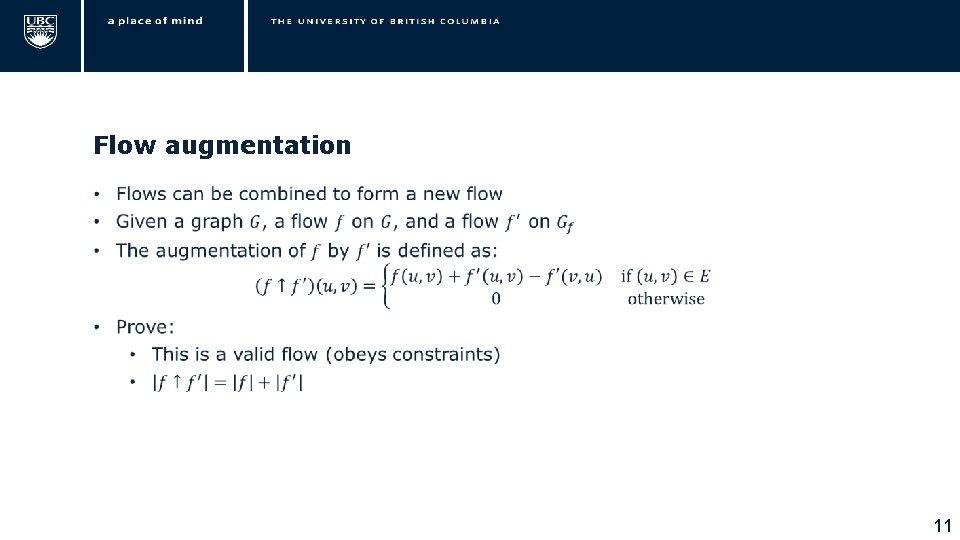Flow augmentation • 11Augmenting path • 12Ford-Fulkerson Algorithm • 13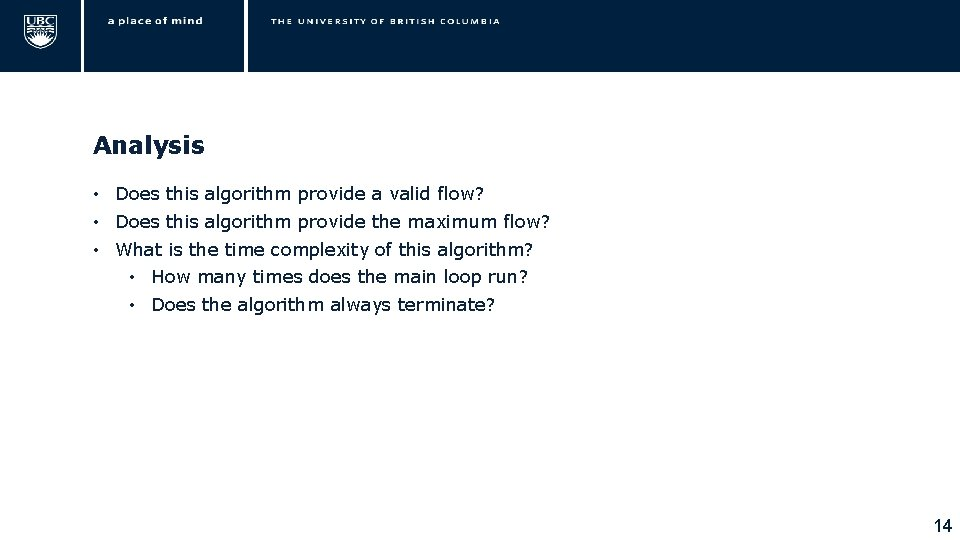Analysis • Does this algorithm provide a valid flow? • Does this algorithm provide the maximum flow? • What is the time complexity of this algorithm? • How many times does the main loop run? • Does the algorithm always terminate? 14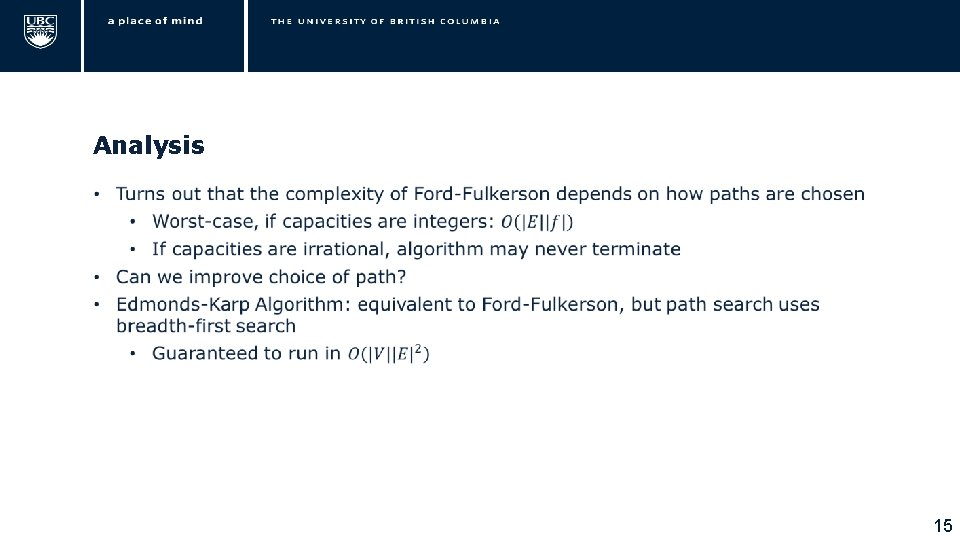Analysis • 15Minimum Cut • • Cut: partition of the nodes of a graph such that two nodes are in different subsets Capacity of the cut: sum of the capacities of all cut edges in one direction Minimum cut: cut with minimum capacity Applications: • Network reliability evaluation 16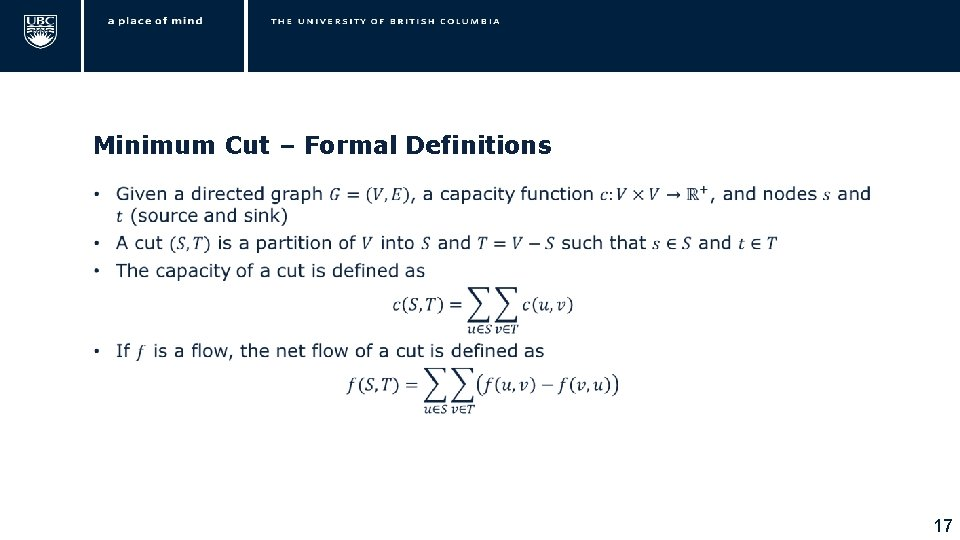Minimum Cut – Formal Definitions • 17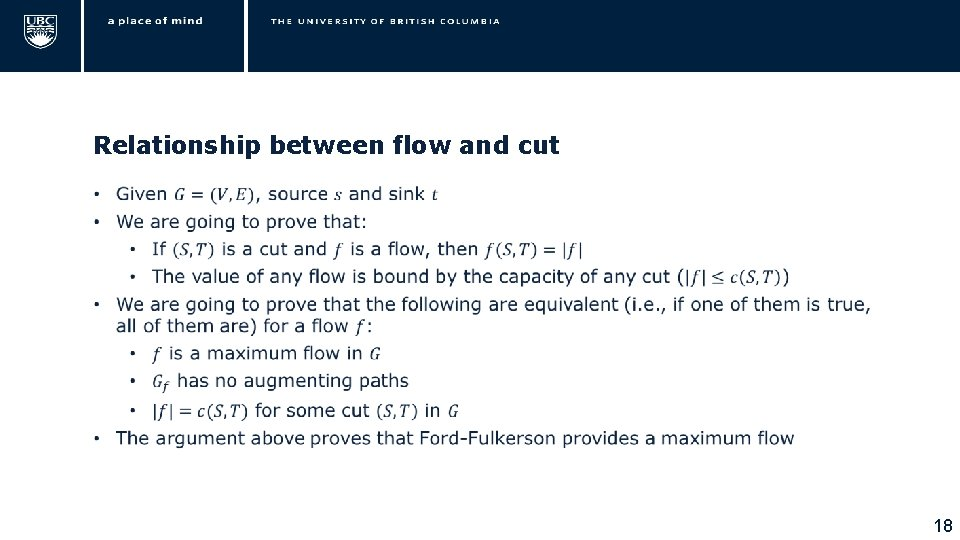Relationship between flow and cut • 18